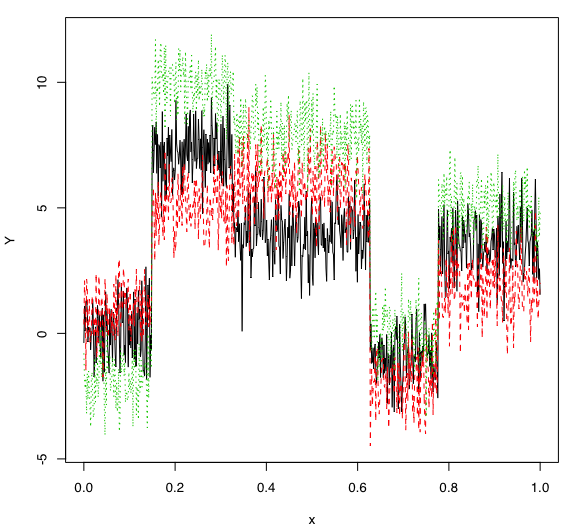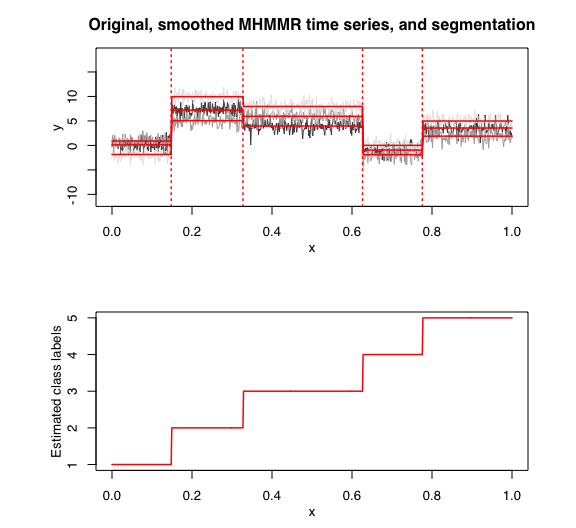# Introduction

In this package, it is possible to select models based on information criteria such as BIC, AIC and ICL.

The selection is done on two parameters which are:

• $$K$$: The number of regimes;
• $$p$$: The order of the polyniomial regression.

# Data

Let’s select a MHMMR model for the following multivariate time series $$Y$$:

data("multivtoydataset")
x <- multivtoydataset$x y <- multivtoydataset[, c("y1", "y2", "y3")] matplot(x, y, type = "l", xlab = "x", ylab = "Y")# Model selection with BIC selectedmhmmr <- selectMHMMR(X = x, Y = y, Kmin = 2, Kmax = 6, pmin = 0, pmax = 3) ## The MHMMR model selected via the "BIC" has K = 5 regimes ## and the order of the polynomial regression is p = 0. ## BIC = -3118.9815385353 ## AIC = -2963.48045745801 The selected model has $$K = 5$$ regimes and the order of the polynomial regression is $$p = 0$$. According to the way $$Y$$ has been generated, these parameters are what we expected. Let’s summarize the selected model: selectedmhmmr$summary()
## ----------------------
## Fitted MHMMR model
## ----------------------
##
## MHMMR model with K = 5 regimes
##
##  log-likelihood nu      AIC       BIC
##        -2894.48 69 -2963.48 -3118.982
##
## Clustering table:
##   1   2   3   4   5
## 100 120 200 100 150
##
##
## ------------------
## Regime 1 (K = 1):
##
## Regression coefficients:
##
##   Beta(d = 1) Beta(d = 2) Beta(d = 3)
## 1   0.1131005   0.9124035   -1.850509
##
## Covariance matrix:
##
##  1.19065699  0.12700417  0.05496662
##  0.12700417  0.90280499 -0.03272115
##  0.05496662 -0.03272115  0.89087804
## ------------------
## Regime 2 (K = 2):
##
## Regression coefficients:
##
##   Beta(d = 1) Beta(d = 2) Beta(d = 3)
## 1    7.190603    5.049411    9.952148
##
## Covariance matrix:
##
##   1.0728107 -0.18105877 0.12234096
##  -0.1810588  1.05365247 0.01237708
##   0.1223410  0.01237708 0.76556432
## ------------------
## Regime 3 (K = 3):
##
## Regression coefficients:
##
##   Beta(d = 1) Beta(d = 2) Beta(d = 3)
## 1    3.951395    5.942001    7.950474
##
## Covariance matrix:
##
##   1.02908516 -0.05875508 -0.02499614
##  -0.05875508  1.19504114  0.02287346
##  -0.02499614  0.02287346  1.01258737
## ------------------
## Regime 4 (K = 4):
##
## Regression coefficients:
##
##   Beta(d = 1) Beta(d = 2) Beta(d = 3)
## 1  -0.9461282   -1.901665   0.0135667
##
## Covariance matrix:
##
##   0.88093255 -0.02771294 -0.03959332
##  -0.02771294  1.14568525 -0.10726848
##  -0.03959332 -0.10726848  0.89326063
## ------------------
## Regime 5 (K = 5):
##
## Regression coefficients:
##
##   Beta(d = 1) Beta(d = 2) Beta(d = 3)
## 1    3.549319    1.888239    4.981038
##
## Covariance matrix:
##
##  1.1333259 0.25852405 0.03091090
##  0.2585241 1.21454178 0.05663565
##  0.0309109 0.05663565 0.84027581
selectedmhmmr\$plot(what = "smoothed")